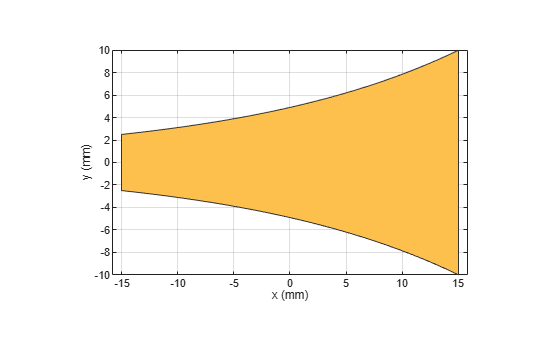# traceTapered

Create tapered trace in X-Y plane

## Description

Use the `traceTapered` object to create a tapered trace in the X-Y plane.

## Creation

### Syntax

``trace = traceTapered``
``trace = traceTapered(Name=Value)``

### Description

example

````trace = traceTapered` creates a tapered trace in the X-Y plane.```

example

````trace = traceTapered(Name=Value)` sets Properties using one or more name-value arguments. For example, `traceTapered(ReferencePoint=[1 1])` creates a tapered trace with the reference point `[1 1]`. Properties not specified retain their default values.```

## Properties

expand all

Name of the tapered trace, specified as a character vector or a string scalar.

Example: `trace = traceTapered(Name="traceTapered")`

Data Types: `char` | `string`

X and Y coordinate of the tapered trace in meters, specified as a two-element vector of real elements.

Example: `trace = traceTapered(ReferencePoint=[1 1])`

Data Types: `double`

Curvature rate of the tapered trace in meters, specified as a real scalar. Set the property to 0 to create a linear trace.

Example: `trace = traceStep(CurvatureRate=20)`

Data Types: `double`

Width of the tapered trace at the input end in meters, specified as a positive scalar

Example: `trace = traceTrapered(Width=0.0060)`

Data Types: `double`

Width of the output side of the taper trace in meters, specified as a positive scalar

Example: `trace = traceTrapered(Width=0.070)`

Data Types: `double`

Length of the tapered trace in meters, specified as a positive scalar.

Example: `trace = traceTrapered(Length=0.040)`

Data Types: `double`

Symmetry of tapered trace along X-axis, specified as `1` or `0`. If set to `1`, symmetry is enabled.

Example: `trace = traceTapered(Symmetry=0)`

Data Types: `logical`

## Object Functions

 `add` Boolean unite operation on two RF PCB shapes `subtract` Boolean subtraction operation on two RF PCB shapes `intersect` Boolean intersection operation on two RF PCB shapes `plus` Shape1 + Shape2 for RF PCB shapes `minus` Shape1 - Shape2 for RF PCB shapes `and` Shape1 & Shape2 for RF PCB shapes `area` Calculate area of RF PCB shape in square meters `rotate` Rotate RF PCB shape about defined axis `rotateX` Rotate RF PCB shape about x-axis `rotateY` Rotate RF PCB shape about y-axis and angle `rotateZ` Rotate RF PCB shape about z-axis `translate` Move RF PCB shape to new location `scale` Change size of RF PCB shape by fixed amount

## Examples

collapse all

Create a tapered trace with default values.

`trace = traceTapered`
```trace = traceTapered with properties: Name: 'mytraceTapered' ReferencePoint: [0 0] CurvatureRate: 50 InputWidth: 0.0050 OutputWidth: 0.0200 Length: 0.0300 Symmetry: 1 ```

Visualize the step trace.

`show(trace)`Create a nonsymmetrical tapered trace.

```trace = traceTapered; trace.Symmetry = 0;```

Rotate by 180 degrees about the Z-axis.

`trace = rotateZ(trace,180);`

Visualize the step trace.

`show(trace)`## Version History

Introduced in R2022a